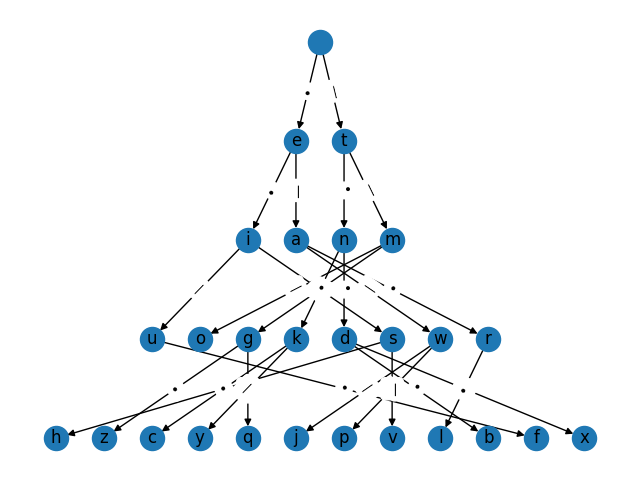# Morse Trie#

A prefix tree (aka a “trie”) representing the Morse encoding of the alphabet. A letter can be encoded by tracing the path from the corresponding node in the tree to the root node, reversing the order of the symbols encountered along the path.```•• •—•• ——— •••— • —• • — •—— ——— •—• —•— —••—
```

```import networkx as nx

# Unicode characters to represent the dots/dashes (or dits/dahs) of Morse code
dot = "•"
dash = "—"

morse_direct_mapping = {
"a": dot + dash,
"b": dash + dot * 3,
"c": dash + dot + dash + dot,
"d": dash + dot * 2,
"e": dot,
"f": dot * 2 + dash + dot,
"g": dash * 2 + dot,
"h": dot * 4,
"i": dot * 2,
"j": dot + dash * 3,
"k": dash + dot + dash,
"l": dot + dash + dot * 2,
"m": dash * 2,
"n": dash + dot,
"o": dash * 3,
"p": dot + dash * 2 + dot,
"q": dash * 2 + dot + dash,
"r": dot + dash + dot,
"s": dot * 3,
"t": dash,
"u": dot * 2 + dash,
"v": dot * 3 + dash,
"w": dot + dash * 2,
"x": dash + dot * 2 + dash,
"y": dash + dot + dash * 2,
"z": dash * 2 + dot * 2,
}

### Manually construct the prefix tree from this mapping

# Some preprocessing: sort the original mapping by code length and character
# value
morse_mapping_sorted = dict(
sorted(morse_direct_mapping.items(), key=lambda item: (len(item), item))
)

# More preprocessing: create the reverse mapping to simplify lookup
reverse_mapping = {v: k for k, v in morse_direct_mapping.items()}
reverse_mapping[""] = ""  # Represent the "root" node with an empty string

# Construct the prefix tree from the sorted mapping
G = nx.DiGraph()
for node, char in morse_mapping_sorted.items():
pred = char[:-1]
# Store the dot/dash relating the two letters as an edge attribute "char"

# For visualization purposes, layout the nodes in topological order
for i, layer in enumerate(nx.topological_generations(G)):
for n in layer:
G.nodes[n]["layer"] = i
pos = nx.multipartite_layout(G, subset_key="layer", align="horizontal")
# Flip the layout so the root node is on top
for k in pos:
pos[k][-1] *= -1

# Visualize the trie
nx.draw(G, pos=pos, with_labels=True)
elabels = {(u, v): l for u, v, l in G.edges(data="char")}
nx.draw_networkx_edge_labels(G, pos, edge_labels=elabels)

# A letter can be encoded by following the path from the given letter (node) to
# the root node
def morse_encode(letter):
pred = next(G.predecessors(letter))  # Each letter has only 1 predecessor
symbol = G[pred][letter]["char"]
if pred != "":
return morse_encode(pred) + symbol  # Traversing the trie in reverse
return symbol

# Verify that the trie encoding is correct
import string

for letter in string.ascii_lowercase:
assert morse_encode(letter) == morse_direct_mapping[letter]

print(" ".join([morse_encode(ltr) for ltr in "ilovenetworkx"]))
```

Total running time of the script: ( 0 minutes 0.182 seconds)

Gallery generated by Sphinx-Gallery